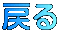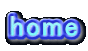\$B2a5n\$N\$*\$9\$9\$a(B

\$B\$3\$l\$^\$G\$K\$*\$9\$9\$a\$G>R2p\$7\$?\$b\$N\$N0lMw\$G\$9!#(B
\$B8=:_!"Gd@Z\$l\$d:_8K@Z\$l\$G:n\$l\$J\$\$%+%/%F%k\$b\$"\$j\$^\$9!#(B

2021\$BG/(B
 \$B%*%j%8%J%k%+%/%F%k(B \$B\$*\$9\$9\$a\$N\$* \$B\$*\$9\$9\$a\$N%+%/%F%k(B 1\$B7n(B (IJ^2?]%50Z(B (IL^W/8%L^Y(B12\$BG/(B (IL^Z2L^%L^Y(B 2\$B7n(B (IZ/D^%=C0<^(B (I50YD^J_0(B12\$BG/(B (I<^]%J^/8(B 3\$B7n(B \$B2V\$"\$+\$j(B (I2A[0=^SYD(B&(I8^Z0](B505 (I:^0YC^]%L(=^(B 4\$B7n(B 5\$B7n(B 6\$B7n(B 7\$B7n(B 8\$B7n(B 9\$B7n(B 10\$B7n(B 11\$B7n(B 12\$B7n(B

2020\$BG/(B
 \$B%*%j%8%J%k%+%/%F%k(B \$B\$*\$9\$9\$a\$N\$* \$B\$*\$9\$9\$a\$N%+%/%F%k(B 1\$B7n(B (I=S0Y%W/D(B (IN_0Y<^[0%8^Z0L_<^-0=(B (I=[0<^]%L(=^(B 2\$B7n(B (IA0C(]8^%J0D(B (IJ2W]D^%<^,0F0(B (I665%L(=^(B 3\$B7n(B (IK^-02]8^%J_0C(0(B (I6EC^(1]%8WL^(B (IJ^Z]<1(B 4\$B7n(B (IF-0%RC^(=](B (I<0J^=X06^Y(B18\$BG/(IP=^EW6=8L(F/<-(B (IN\2D%ZC^((B 5\$B7n(B (IL^W2D%E2D(B (I1OJ6^]\$B-b(B2 (I6XL+YF1%ZSH0D^(B 6\$B7n(B (IK^-3^*0Y(B (IJ0DW]D^K^0Y(B (ILZ]A(B75 7\$B7n(B (IB<^D^3(B10\$B!A(IZ>/<.]\$B!A(B (I8^Z]L(C^(/8(B12\$BG/(B (ID0=C/D^%10S]D^(B 8\$B7n(B (I?Y%C^%M^W0I(B (I8^Z]S0Z]<^(B18\$BG/(B (I=L_S0F(B 9\$B7n(B (I1R<^=D(B (IN^3S1(B12\$BG/(B (I7Y<-%6<=(B 10\$B7n(B (I<^*HWY%E/B(B (I:03^'Y\$B!!(I<]8^YJ^ZY\$B!!(IJ^0N^](B (I32=70%O/8(B 11\$B7n(B (I (IF/6\$B!!(I>/<.](B (I:^0YC^]%D^X0Q(B 12\$B7n(B (I;2Z]D%P/D^E2D(B (IK^/8^K_0D8X=O=4C^(<.](B2020 (I70Y%[\2TY(B

2019\$BG/(B
 \$B%*%j%8%J%k%+%/%F%k(B \$B\$*\$9\$9\$a\$N\$* \$B\$*\$9\$9\$a\$N%+%/%F%k(B 1\$B7n(B (IM_5F0%N^1(B (IJ^W]@2](B17\$BG/(IXPC/D^4C^(<.](B (IK_-1%WL^(B 2\$B7n(B (IL^Z=(B \$B0KC#(B (IPX5]%@^W0(B 3\$B7n(B (I40Y(B (I;]DX0%[0TY(B (I[2TY%L(=^(B 4\$B7n(B (IM2>2(B (I10D^S1\$B!!(IZ6^<0(B (IK_]8%J^2?](B 5\$B7n(B (IZ2\(B (I;^%F/6\$B!!(IC0W0D^(B (I<]6^N_0Y%=X]8^(B 6\$B7n(B (I2O<^](B (IN_0Y%<^.]\$B!!(IL^XX1]=(B (I<^.]%:X]=^(B 7\$B7n(B (IB<^D^3(B9\$B!A(IP=C(%E2D\$B!A(B (IJ_]M_[\$B!!(I1FM^Y;X5(B (I=[0%C70W(B 8\$B7n(B (I;O0=62(B (I8^Z]S0Z]<^7]@%YJ^](B (IJJ^E%K^0A(B 9\$B7n(B (II0;2D^(B (IA];^I\$B!!(I5W]A.(B (I:]A0@(B 10\$B7n(B (I:];]L_<.](B \$B6A(IL^Z]@^0=^%A.2=(B (I6<=%?0@^(B 11\$B7n(B (IK^8DX0[0D^(B \$BJK(B (I6Y3^'D^=%68CY(B 12\$B7n(B (I@K_56%A,A,%50Z(B (IK^/8^K_0D8X=O=(B2019 (I1/L_Y60(B

2018\$BG/(B
 \$B%*%j%8%J%k%+%/%F%k(B \$B\$*\$9\$9\$a\$N\$* \$B\$*\$9\$9\$a\$N%+%/%F%k(B 1\$B7n(B ff\$B!J(IL+YC( (I=6X0\8^(B (IL^YD^/8^%J2N^0Y(B 2\$B7n(B \$BJ4@c(B (IJ^W]@2]\$B!!(I<]8^YSYD\$B!!(I8^Z]J^7^0(B15\$BG/(B \$B@c9q(B 3\$B7n(B (IA,0P](B (IJ2W]D^J_08\$B!!(I@^085X<^]=^(B (I;8W%<^]<^,0(B 4\$B7n(B (I=L_X]8^%?]8^(B (IS]70<.Y@^0(B (I=0=^%DF/8(B 5\$B7n(B (IM^=D%C^2(B (I<^.F03+060\$B!!(IL^W/8WM^Y(B12\$BG/(B (I1W3]D^%;^%\0YD^(B 6\$B7n(B (I=:0Y(B (I6HOW(B (I5Z]<^%L^[/;Q(B 7\$B7n(B (IB<^D^3(B8\$B!A=cNx2N!A(B (I8^Z]S0Z]<^5X<^EY(B (I<^]%L(=^(B 8\$B7n(B (I1C^X0G(B (IZS]J0D%C^RWW(B (IJ_W@^2=(B 9\$B7n(B (I8Z/>]D(B (I8^Z]D^[E/8(B12\$BG/(B (I>L_C]J^0%S0](B 10\$B7n(B (IDWS]D(B (I208^Y%Z1(B10\$BG/(B (IL^W/8%Y<1](B 11\$B7n(B (I\];2D^%WL^(B (IN_0D<,0[/D(B10\$BG/(B (I1L@0%C^(E0(B 12\$B7n(B (I32]@0%L']@<^0(B (IK^/8^K_0D8X=O=J^0<^.](B2018 (IOP0%C2W0(B

2017\$BG/(B
 \$B%*%j%8%J%k%+%/%F%k(B \$B\$*\$9\$9\$a\$N\$* \$B\$*\$9\$9\$a\$N%+%/%F%k(B 1\$B7n(B (I:^/D^J^0D^(B (I\2YD^@070\$B!!(IX1L^X0D^(B (IL^Y0J^0D^(B 2\$B7n(B (I=I0L']@<^0(B \$B5(\$NH~!!5~ET(ID^W2<^](B (IN^=D]80W0(B 3\$B7n(B (IWL^@^]=(B (I8W2GX/<-(B14\$BG/(B (IPS;^(B 4\$B7n(B (I342D%=L_X]8^(B (I<^,/8%@^F4Y\$B!!(I<]8^YJ^ZY\$B!!(BThe Yokohama (I2=W%C^%K_I=(B 5\$B7n(B (I8^X0]%8^X0]^(B (I8^Z]S0Z]<^\$B!!(IJ^6Y@(B (I4J^0%8^X0](B 6\$B7n(B (IZ2]%@^]=(B \$B;3:j(IXPC/D^%4C^(<.](B2017 (I32=70%P=D(B 7\$B7n(B (IB<^D^3(B7\$B!A(I9^/D%\2YD^\$B!A(B (I10D^M^/8^\$B!!(I9YK_0(B (IR7<6](B 8\$B7n(B \$B??2F\$N2L (IC^([]\$B!!(IDZ3^-0WQ\$B#V#S#O#P(B (I4RWYD^%80W0(B 9\$B7n(B (I50@Q%;2](B (IJ^Y3^*F0(B14\$BG/(I6XK^1]6=8(B (I12=%L^Z060(B 10\$B7n(B (IW2<^]8^%32]D^(B (I;^%O/6W]\$B!!(I@^L^Y6=8(B12\$BG/(B (IO[]%N/J_0(B 11\$B7n(B (IK^V]D^%;^%E2D=62(B (IN_0Y<^[0(B15\$BG/(B (IH8^[0F(B 12\$B7n(B (IRX8X(B (IK^/8^%K_0D8X=O=J^0<^.](B2017 (I=Z/<^%J]O0(B

2016\$BG/(B
 \$B%*%j%8%J%k%+%/%F%k(B \$B\$*\$9\$9\$a\$N\$* \$B\$*\$9\$9\$a\$N%+%/%F%k(B 1\$B7n(B (I;Y0(B (IM^]X1/8(B12\$BG/(I<*X03/D^(B (IJ^EE%L^X=(B 2\$B7n(B (I<.:8W(B (I8^Z]L(C^(/8(B18\$BG/(I=S0YJ^/AX;^0L^(B (IN/D%32=70%DC^0(B 3\$B7n(B (IC^(R]<1(B (IJ^W]@2](B17\$BG/(I=7,J_4C^(<.](B (IS/7]J^0D^(B 4\$B7n(B (I:OA=^%=O2Y(B (IL^/<-PY=^<]8^YSYD(B16\$BG/(B (IA,0X0%A,/L_X](B 5\$B7n(B \$B70Iw(B \$BIY;N;3O<(IL^Z]C^/D^(B18\$BG/(B (IO@D^0Y(B 6\$B7n(B \$B;gM[2V(B \$B;3:j(IXPC/D^%4C^(<.](B2016 (IA,2E%L^Y0(B 7\$B7n(B (IB<^D^3(B6\$B!A(IK^0A%@2Q\$B!A(B (I10D^M^/8^\$B!!(I@^08%:03^(B (IJ_[O(B 8\$B7n(B (IX5%60(B \$B6A(B17\$BG/(B (I@^27X(B 9\$B7n(B (I>L_C]J^0%Z2](B (IJ^0M^2D%L^1Y(B10\$BG/(B (IS]DF/8(B 10\$B7n(B (I8^/C^0(B \$B%i%C%-!<%-%c%C%H!!#A#s#h(B99 (IN^/A%N^0Y(B 11\$B7n(B (IC^(0L_%50@Q(B (I58DS1(B07.1(I=:C(/<-J^0Z2(B (IHJ^@^(B 12\$B7n(B (II4Y(B (I<^,/8%@^F4Y\$BAO6H(B150\$B<~G/(B (IN/D%2@X1](B

2015\$BG/(B
 \$B%*%j%8%J%k%+%/%F%k(B \$B\$*\$9\$9\$a\$N\$* \$B\$*\$9\$9\$a\$N%+%/%F%k(B 1\$B7n(B (I<.0](B (I:Y:Y(B (IJ^6YC^((B 2\$B7n(B \$BK:\$l@c(B (I<,D0%D^%L^Y2U(B15\$BG/(B (IJ_J_9^0E(B 3\$B7n(B (IXX:2(B (IIL^8X08%<]8^YJ^ZY(B (I6P6>^(B 4\$B7n(B \$B=U>,(B \$B6A(I<^,J_F0=^J0SF0(B (IJ^]L^0(B 5\$B7n(B (IY%3^']M^0Y(B (I8^Z]L(C^(/8(B18\$BG/(B (I7-0J^%XL^Z(B 6\$B7n(B (IOF,6^J(B (I10D^M^/8(B10\$BG/(B (I=62%@^2K^]8^(B 7\$B7n(B (IB<^D^3(B5\$B!A(I163W\$B!A(B (I3/D^L+0D^%X;^0L^(B (I6^YL=DX0Q(B 8\$B7n(B (IWF191(B (I16^J^Z=%ZN_;D^(B (I<^]%X/70(B 9\$B7n(B (I9A0C(B \$BCNB?(B (I1L_X:/D%80W0(B 10\$B7n(B (I:IP0(B (IW/707,/D(B (I90L_:/@^0(B 11\$B7n(B (IK2WF(B (I;]<^*YO](B (IL'<^0%H0L^Y(B 12\$B7n(B (IM_(B (I8YN^1<^*%48=DWJ^6^]=(B (I<,]J_]%68CY(B

2014\$BG/(B
 \$B%*%j%8%J%k%+%/%F%k(B \$B\$*\$9\$9\$a\$N\$* \$B\$*\$9\$9\$a\$N%+%/%F%k(B 1\$B7n(B (I5YL*0L^Y(B (IN\2DN0=(B12\$BG/(B (IC70W%;]W2=^(B 2\$B7n(B (IN^]N^](B (I<,D0D^%L^Y2U(B (I1Z7;]@^0(B 3\$B7n(B \$BBT\$A:y(B \$BGr=#(I<*X06=8(B2014\$B!!(B (I<^]%DF/8(B 4\$B7n(B \$B2Z:i(B \$BC]Da(IK_-1SYD(B (IJ^25Z/D%L(=^(B 5\$B7n(B (I:O]@ZL^0(B (I2A[0=^SYD%<^.060(B (IP]D%<^-Z/L_(B 6\$B7n(B (I<^,J_]%L^Y0(B \$B;3:j(IXPC/D^4C^(<.](B2014 (I62K_X0F,(B 7\$B7n(B (IB<^D^3(B4\$B!A3\$4_J*8l!A(B (IF/6%6L*%SYD(B (IOY6^X0@(B 8\$B7n(B (I;O0%;=K_<.](B (ID^]%LX5\$B!!(I1HN(B (IK_F,%:W0@^(B 9\$B7n(B (IN^/94(B (IJ^W]@2](B17\$BG/!!(I8^Z]L'08W=4C^(<.](B (I8[]@^28%J2N^0Y(B 10\$B7n(B (I=20B%O[](B (I;^%F/6(B12\$BG/(B (I@^0<^X]%80W0(B 11\$B7n(B (IEF62(B (I1]I/8(B12\$BG/(B (I246^0%DF/8(B 12\$B7n(B (IJ^/8%D)%C^(>]J^0(B \$BC]Da(B21\$BG/(B (I70Y%2]M_X1Y(B

2013\$BG/(B
 \$B%*%j%8%J%k%+%/%F%k(B \$B\$*\$9\$9\$a\$N\$* \$B\$*\$9\$9\$a\$N%+%/%F%k(B 1\$B7n(B (I=H08%12=^(B (IP/<*Y%U10Y(B (I7^QZ/D(B 2\$B7n(B (IL'0=D%7=(B (I=^L^[/6(B (I7=%5L^%L'210(B 3\$B7n(B \$B>EFn(I=D0X0(B \$BGr=#(I<*X06=8(B2013 (IA*X0%L^[/;Q(B 4\$B7n(B (IF-0<0=^](B (IJ^/L'[0\$B!&(IDZ2=(B (IDQ%:X]=^(B 5\$B7n(B \$B?7NP(B \$B;3:j(IMK^X0K_0C/D^(B (I<^,/8%[0=^(B 6\$B7n(B (I<]8^(B (I8^Z]8^W]D(B10\$BG/(B (ISK0D(B 7\$B7n(B (IB<^D^3\$B!!(IJ_0D(B3 (I2Y%D^%Z(B (I<0L^X0=^(B 8\$B7n(B (I13^*]@D^0Y(B (IF/6%6L*%8^Z0](B (IO2@2(B 9\$B7n(B (I[0D^8[;2D(B (IJ^W]@2](B17\$BG/!!(IPYD]@^L4C^(<.](B (I?Y8J^0I(B 10\$B7n(B (IN^MP1](B (I<0J^=X06^Y\$B!!(IP=^EW(B (I:=SN_X@](B 11\$B7n(B (I4YK_=(B \$B6A(B17\$BG/(IR[0J0SF0(B (I:^/D^L'0;^0(B 12\$B7n(B (IRX0%RX0(B \$B5\>k6.(B15\$BG/(B (I70Y(B

2012\$BG/(B
 \$B%*%j%8%J%k%+%/%F%k(B \$B\$*\$9\$9\$a\$N\$* \$B\$*\$9\$9\$a\$N%+%/%F%k(B 1\$B7n(B (ID^W:^]=^%J_Z=^(B (I[];6J_(B (I>]CEX5(B 23\$BG/(B (IN/D%J^@0D^%WQ(B 2\$B7n(B (I1D^[(B (IL^EJ0L^](B12\$BG/(B (IL^Y<./D(B 3\$B7n(B (IXJ^2J^0(B (I<^Q%K^0Q(B (I?YC(%D^/8^(B 4\$B7n(B (I=L_X]8^%K^<^/D(B (I7YN0O](B3\$BG/(B (I=8X-0D^W2J^0(B 5\$B7n(B (I15?^W(B (IN_YL(C^(5\$B!!(I1HN(B (IV:JO(B 6\$B7n(B (I=O2Y(B \$BC]Da(B17\$BG/(B (I34C^(]8^%M^Y(B 7\$B7n(B (IS]70%O<^/8(B \$BGr=#(IMK^X0K_0C/D^(B (IL^Y0%J\2(B 8\$B7n(B (I<,]8^0J2(B (I8^Z]S0Z]<^\$B!!(I1YC2](B (I5X]K_/8(B 9\$B7n(B (I507/D^(B (I2A[0=^SYD\$BCaIc(I;^%L[10SYC/D^(B (I6]J_X%?0@^(B 10\$B7n(B (I50@Q%X0L^=(B (IN_0Y<^[0(B25\$BG/(B (IZ/D^12(B 11\$B7n(B (I6RTO(B (II10=^PY(B (IO]J/@](B 12\$B7n(B (IN^]D^%6^0Y(B (IL^Y6^Y(B1888 XYZ

2011\$BG/(B
 \$B%*%j%8%J%k%+%/%F%k(B \$B\$*\$9\$9\$a\$N\$* \$B\$*\$9\$9\$a\$N%+%/%F%k(B 8\$B7n(B (IL_[[08^(B \$BGr=#(B12\$BG/(B 9\$B7n(B (IN_0D:0Y(B (IZ5N_YD^%8^YRY(B (IL_YP4Y%;L^0Y(B (IL^W/C^(%R1X0(B 10\$B7n(B (I74]%>W(B (I;^%L_ZP1Q%SYB(B (IS=:%P-0Y(B 11\$B7n(B (IB<^D^3(B \$B;3:j(B12\$BG/(B (IC(5M_M_(B&(IDF/8(B 12\$B7n(B (IB<^1](B (IWL[28^(B10\$BG/(B (I;2D^60(B